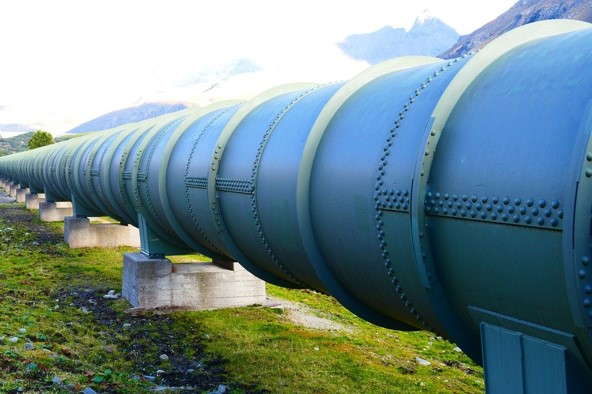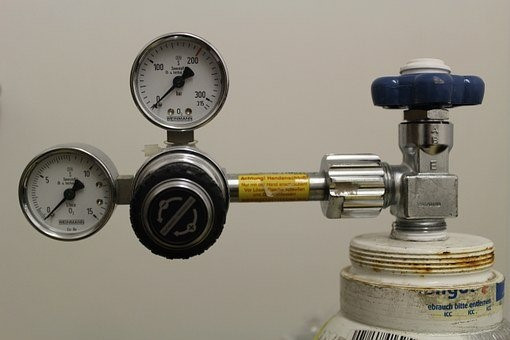# 13 Calculating Grade or Length

Click play on the following audio player to listen along as you read this section.So far in this chapter we’ve gone through the motions to end up finding the total fall on an object given its length and grade. This will work well for us in all kinds of situations in the field.

But from a math perspective we should also be able to rework the formula to solve for length or grade.

Let’s start by just putting up the total fall formula once again.

$\text{Total fall} = \text{length}\times\text{grade}$

Instructors and students quite often use a triangle to solve for the variables in a formula when there are three variables in that formula. As we have three variables in the total fall formula why don’t we go ahead and use this method. Check out the drawing below to get an idea of what I’m talking about.

From this triangle we can get all three versions of the formula.

That’s the magic of the mathematical triangle. It can help you solve for any of the variables in a formula with three variables. If you ever run across other formulas with three variables give it a try. In fact the next chapter deals with trigonometry and you can use this triangle method to solve for a lot of stuff.

As we’ve already talked about and solved problems for total grade we’ll just stick to the other two variables. We’ll do a couple examples solving for length and a couple solving for grade.

# Solving for Length

$\text{length } = \dfrac{\text{total fall}}{\text{grade}}$

We’ll do examples using each of the three types of grade.

ExamplePipe is run where the total fall from one side to the other is 0.59 meters. The grade on the pipe is a ratio of 1 in 50. What length of pipe must be run in order to achieve this total fall.

Step 1: Write down the formula you’ll be working with.

$\text{length } = \dfrac{\text{total fall}}{\text{grade}}$

Step 2: Write down the variables making sure they are in units you can work with.

$\text{total fall: } 0.59 \text{ meters}$

$\text{grade: } 1/50$

Step 3: Plug in the variables and solve.

$\text{length} = \dfrac{0.59}{\dfrac{1}{50}}$

Mathematically this is the same as saying point five nine multiplied by fifty.

$\begin{array}{c} \text{length} &= 0.59 \times 50 \\ \text{length} &= 29.5 \text{ meters} \end{array}$

ExamplePipe for a compressed air system is run with a grade of 1%. The total fall on the pipe from one side of a building to the other side of a building is 17 inches. What is the length of pipe that has been run.

Step 1: Write down the formula you’ll be working with.

$\text{length } = \dfrac{\text{total fall}}{\text{grade}}$

Step 2: Write down the variables making sure they are in units you can work with.

$\text{total fall: } 17 \text{ inches}$

$\text{grade: } 1\% \text{ or }0.01$

Step 3: Plug in the variables and solve.

$\begin{equation}\begin{split} \text{length} &= \dfrac{17}{0.01} \\ \text{length} &= 1700 \text{ inches} \end{split}\end{equation}$Remember that when dealing with percent the total fall and the length will be in similar units. As I wrote this I originally put in the length as 1700 feet. This is an easy mistake to make and one to really look out for.

ExamplePipe is run in a parkade and the total fall from one side to the other is 1 foot 3 inches. The grade on the pipe is 1/8″ per foot. How far does the pipe go at that grade?

Step 1: Write down the formula you’ll be working with.

$\text{length } = \dfrac{\text{total fall}}{\text{grade}}$

Step 2: Write down the variables making sure they are in units you can work with.

$\text{total fall: } 1 \text{ foot } 3 \text{ inches}$

$\text{grade: } 1/8"/ft$

Note that when dealing with the grade in inches per foot the total fall must be in inches. Therefore the 1 foot 3 inches must be changed to 15 inches.

$\text{total fall: } 15 \text{ inches}$

Step 3: Plug in the variables and solve.

$\begin{equation}\begin{split} \text{length} &= \dfrac{15}{1/8"/\text{foot}} \\ \text{length} &= 120 \text{ feet}\end{split}\end{equation}$

Once again remember that when using the total fall formula with inches per foot as the grade the length always ends up being in feet.This might actually get a bit trickier than just solving for length.

When finding the grade the units really come into play. Remember that for both percent and a ratio there are actually no units in the grade. This means that when solving the problems we must make sure to cancel out units to leave us with none. In the end its important when finding those two grades that both the length and the total fall are in the same units. When I go through the examples just below I’ll show you what I mean.

When using inches per foot it’s also important to note what the units are in. Remember that the grade must end up being in inches per foot. This means that the length must be in feet while the total fall must be in inches. If this is not the case then the variables must be changed to match this.

Let’s head straight to some examples so you can see what I’m talking about.

ExamplePipe is run where the total fall from one side to the other is 0.59 meters. The length of the pipe is 29.5 meters. What is the grade expressed as a ratio over 50?

Step 1: Write down the formula you’ll be working with.

$\text{grade} = \dfrac{\text{total fall}}{\text{length}}$

Step 2: Write down the variables making sure they are in units you can work with.

$\text{total fall: } 0.59 \text{ meters}$

$\text{length: }29.5 \text{ meters}$Note that both variables are in the same units. This works.

Step 3: Plug in the variables and solve.

$\begin{equation}\begin{split} \text{grade} &= \dfrac{0.59}{29.5} \\ \text{grade} &= 0.02 \end{split}\end{equation}$

Our final goal is to get the grade to a ratio of 1:50. The question is how do we go about getting to that. Follow the math here as this is where it gets a bit tricky.

Start off by putting the answer into a ratio over 1.

$\begin{equation}\begin{split} \text{grade} &= 0.02 \\ \text{grade} &= \dfrac{0.02}{1} \end{split}\end{equation}$

Now take that ratio over 1 and get it into a ratio over 50. We do this by multiplying both the top and bottom by 50.

$\begin{equation}\begin{split} \text{grade} &= \dfrac{0.02}{1} \times \dfrac{50}{50} \\ \text{grade} &= \dfrac{1}{50} \end{split}\end{equation}$

There you have it.

ExamplePipe for a compressed air system has a length of 141 feet 8 inches. The total fall on the pipe from one side of a building to the other side of a building is 17 inches. What percent grade is the pipe run at?

Step 1: Write down the formula you’ll be working with.

$\text{grade} = \dfrac{\text{total fall}}{\text{length}}$

Step 2: Write down the variables making sure they are in units you can work with.

$\text{total fall: } 17 \text{ inches}$

$\text{length: }141 \text{ feet } 8 \text{ inches}$

So we have a slight problem here. The total fall is in inches while the length is in feet and inches. We have to make them both the same units. What we’ll do here is change the 141 feet and turn it into inches and then add the 8 inches.

$\begin{equation}\begin{split} 141 \text{ feet} \times 12 in/ft &= 1692 \text{ inches} \\ 1692 \text{ inches} + 8\text{ inches} &= 1700 \text{ inches}\end{split}\end{equation}$

Step 3: Plug in the variables and solve.

$\begin{equation}\begin{split} \text{grade} &= \dfrac{17}{1700} \\ \text{grade} &= 0.01 \end{split}\end{equation}$

Step 4: Change the decimal into a percent by multiplying by 100.

$0.01 \times 100 = 1\%$

ExamplePipe is run in a parkade and the total fall from one side to the other is 1 foot 3 inches. The total length of pipe is 120 feet. What is the grade on the pipe in inches per foot. Put your answer to the nearest eighth of a foot.

Step 1: Write down the formula you’ll be working with.

$\text{grade} = \dfrac{\text{total fall}}{\text{length}}$

Step 2: Write down the variables making sure they are in units you can work with.

$\text{total fall: } 1 \text{ foot} 3 \text{ inches}$

$\text{length: }120 \text{ feet}$

In this case the length is good but the total fall must be in inches for this version of the formula to work. One foot 3 inches is equal to 15 inches.

$\text{total fall: }15 \text{ inches}$

Step 3: Plug in the variables and solve. Remember that the grade has to be in inches per foot so we need to divide each part of the equation by 120.

$\text{grade} = \dfrac{15 \text{ inches}}{120 \text{ feet}} \div \dfrac{120}{120} = \dfrac{0.125 \text{ inches}}{1 \text{ foot}}$

We’re almost there. To turn the 0.125 inches into eights of an inch we multiply the 0.125 by 8.

$0.125 \times 8 = 1$

This represents 1/8 inch and in the end the grade is 1/8 of an inch per foot.

$1/8" \text{ per foot}$

Okay now its your turn to give it a shot. Try the practice questions and check out the video answers when you are done.

# Practice Questions

Question 1Don is running sprinkler lines in the underground parkade for a 30 story office building in downtown Vancouver. His task is to run a sprinkler line from one side of the parkade to the other. If the sprinkler line is graded at 1/125 and the total fall does not exceed 0.5 meters then the sprinkler line will end up running from one side of the parkade to the other. What is the length of the sprinkler pipe?

Question 2

Rachel runs her own plumbing company. She’s been asked to run an underground storm drainage line from one catch basin to a catch basin located at the other end of the property. The grade can not exceed 2% and the total fall on the pipe cannot exceed 25 inches. What is the maximum length the pipe can run in feet and inches?

Question 3Chad just bought himself a farm in Alberta and needs to run some drainage pipe from one barn to the other. The length of the pipe will be 200 feet and the total fall on the pipe will be 24 inches. What will the grade be in order for those numbers to work? Put your answer as a percentage.